## Introduction

We assume you went through the previous vignettes, especially the getting started vignette. In this vignette, you will learn how to use the update_diagrm() function to further customize the look of your diagrams.

To re-cap, the workflow discussed in the previous vignettes goes like this

#specify model
sirmodel1 = list(variables = c("S","I","R"),
flows     = list(S_flows = c("-b*S*I"),
I_flows = c("b*S*I","-g*I"),
R_flows = c("g*I")))
#optional layout information
sirsettings1 = list(varlocations = matrix(data = c("S", "","R",
"", "I","" ),
nrow = 2, ncol = 3, byrow = TRUE),
varbox_x_size = c(1, 1.5, 1),
varbox_y_size = c(2, 1, 1),
varspace_x_size = c(1.5,2),
varspace_y_size = 0.5
)
#prepare diagram
diagram_list1 <- prepare_diagram(model_list = sirmodel1, model_settings = sirsettings1)
#make diagram
sir_diagram1 <- make_diagram(diagram_list = diagram_list1)
#plot diagram
plot(sir_diagram1)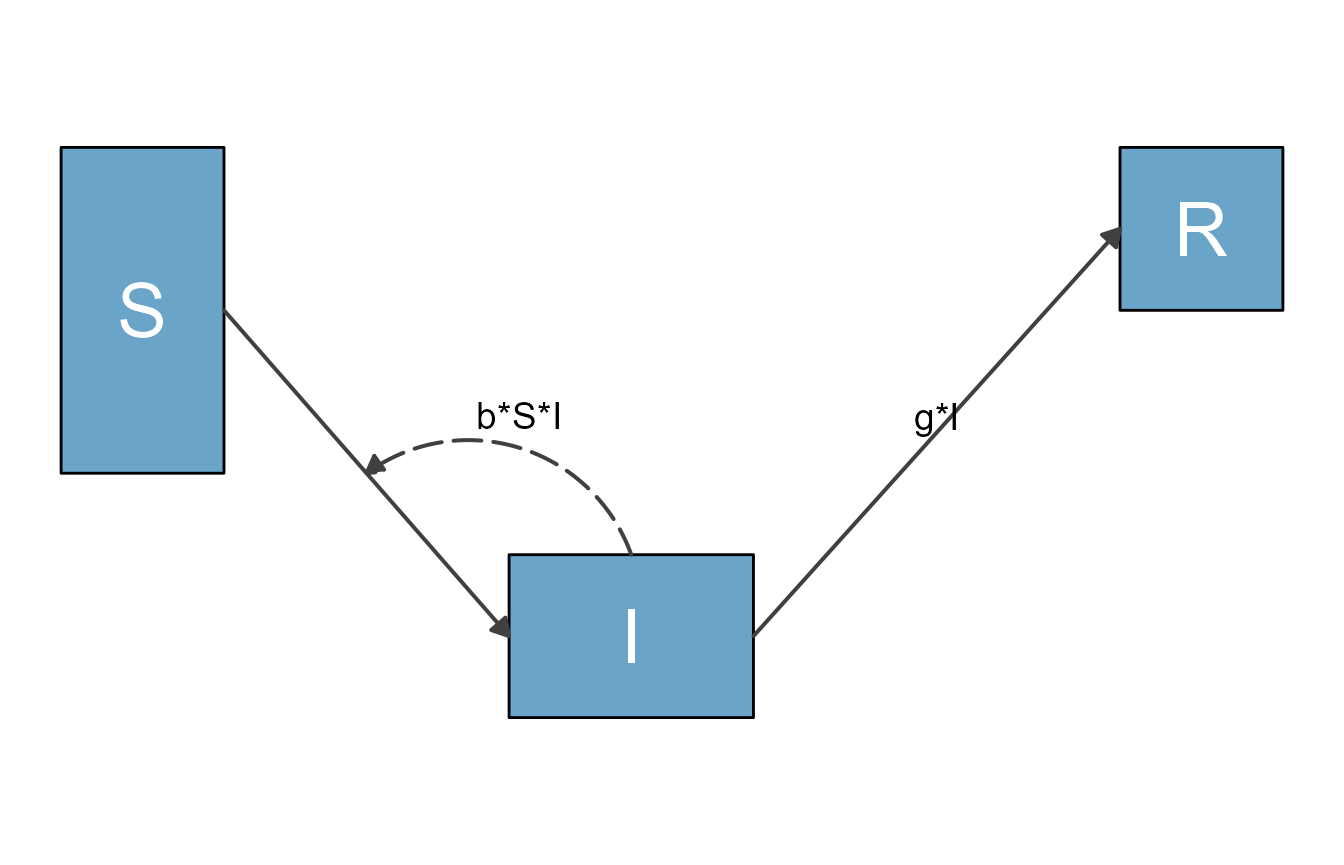## A quick look behind the scenes

Before we look at examples, let’s briefly discuss some aspects of what’s going on behind the scenes.

The function prepare_diagram() takes the model and optional settings and returns a list (which we call diagram_list here) consisting of 2 data frames, one called variables and one called flows. In each data frame, the elements (variables or flows) are in the rows, and the columns indicate graphical settings, e.g. the color. You can look at the diagram_list data frames. Here are those two data frames for the model above:

print(diagram_list1$variables) #> id name xmin xmax ymin ymax xlabel ylabel outline_color fill_color label_text #> 1 1 S 0.0 1 1.5 3.5 0.50 2.5 black #6aa4c8 S #> 2 2 I 2.5 4 1.0 2.0 3.25 1.5 black #6aa4c8 I #> 3 3 R 6.0 7 2.5 3.5 6.50 3.0 black #6aa4c8 R #> label_color label_size #> 1 white 10 #> 2 white 10 #> 3 white 10 print(diagram_list1$flows)
#>   id  name        type from   to xstart xend ystart yend xlabel ylabel
#> 1  1  m_gI        main    I    R   4.00 6.00    1.5  3.0   5.00  2.350
#> 2  2 m_bSI        main    S    I   1.00 2.50    2.5  1.5   1.75  2.100
#> 3  3 i_bSI interaction    I <NA>   3.25 1.75    2.0  2.0   2.50  2.575
#>   curvature line_color line_size line_type label_text label_color label_size
#> 1       0.0     grey25       0.7     solid        g*I       black          5
#> 2       0.0     grey25       0.7     solid                  black          5
#> 3       0.5     grey25       0.7  longdash      b*S*I       black          5
#>   show_label arrow_size show_arrow
#> 1       TRUE       0.25       TRUE
#> 2       TRUE       0.25       TRUE
#> 3       TRUE       0.25       TRUE

When flowdiagramr prepares the diagram, it groups flows into 3 different categories as follows:

• Main flows, which are flows that go from one box to another, and generally indicate physical/actual movement of units from one state to another (e.g., from susceptible to infected).
• Interaction flows, which indicate a variable interacting with another, without any actual flow (e.g., infected individuals infecting susceptible).
• External flows, which indicate entry into or exit out of the system, e.g., births and deaths to/from states that are not part of the model. (The model above has no external flows.)

Each of those flow types receives its own default styling. You can change that as you like. It is also quite possible that sometimes flowdiagramr doesn’t guess correctly and labels a flow differently than what it should be. In that case, you can also easily overwrite the default settings.

In general, before you make the diagram with make_diagram(), you can adjust all the settings for each variable/box or flow/arrow. A direct way is to just edit the elements of the two data frames by hand. For instance this code changes the color of the box for the I variable to red and increases the size of the interaction flow showing the infection process.

#make a copy
diagram_list2 <- diagram_list1
#change some styling
diagram_list2$variables$fill_color <- "red"
diagram_list2$flows$line_size <- 1.5

This is how the updated diagram looks.

sir_diagram2 <- make_diagram(diagram_list2)
plot(sir_diagram2)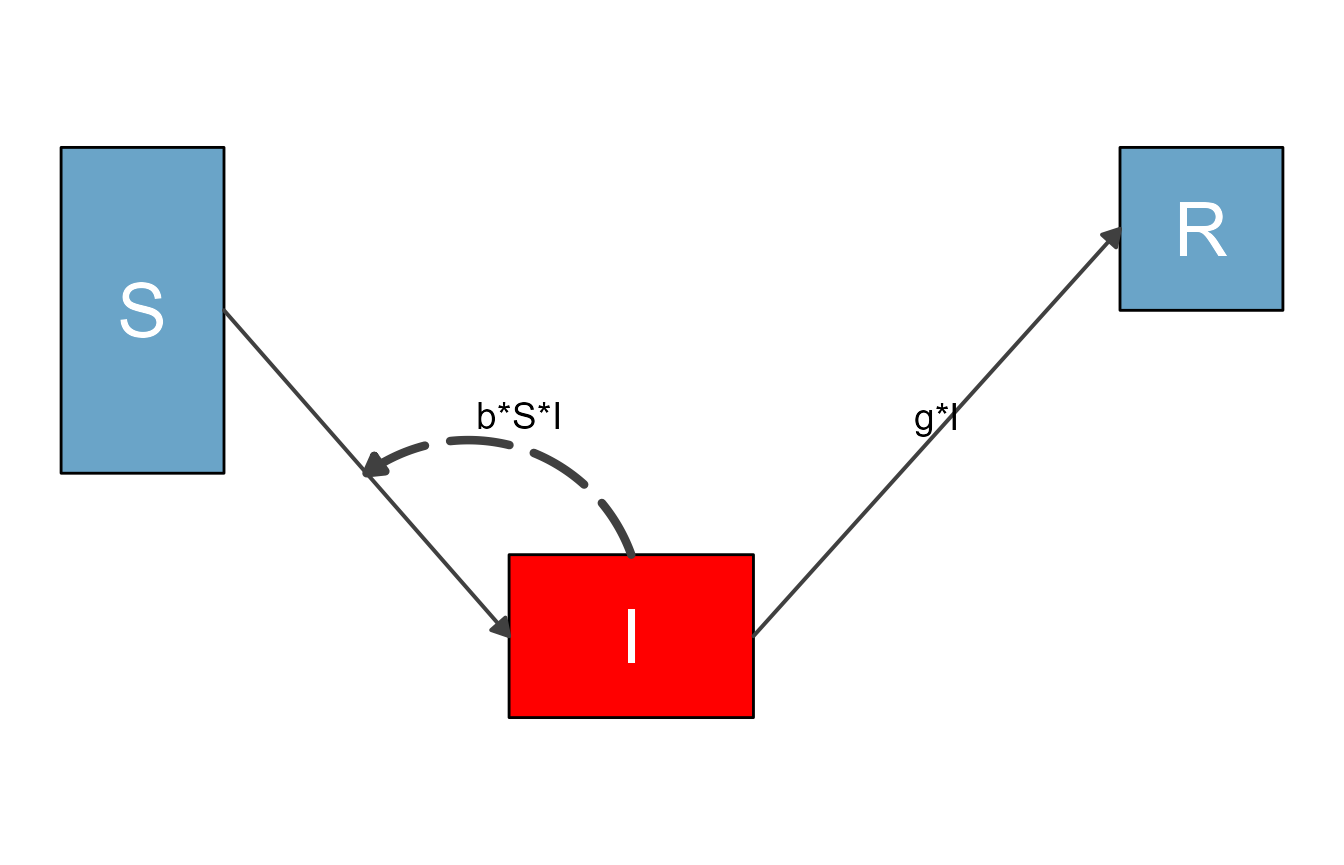This way of manually updating is always possible, but it is a bit tedious. To make updating the look of the various plot elements easier, flowdiagramr contains a convenience function called update_diagram(), which simply does the updating of the elements in either of those data frames based on the supplied input. The function needs two inputs. The first input is the list returned by prepare_diagram(). The second input is a list of named entries which specify what elements of the diagram you want to update. The help file for update_diagram() provides all the details for allowed inputs. The examples we explore now show several of the options.

## Example 1

We’ll start by repeating the changes we made above, now using update_diagram().

To update specific elements (variables or flows), you need to provide their names, as you will see below. You can get the names of all elements by calling update_diagram() with only the diagram_list element, like so:

update_diagram(diagram_list1)
#> No settings were provided; returning names of elements in diagram_list dataframes.
#> Variables: S, I, R
#> Flows: m_gI, m_bSI, i_bSI

If you wanted to make the same changes as we did manually above, this would do it

diagram_list3 <- update_diagram(diagram_list1,
diagram_settings = list(var_fill_color = c(I = "red"),
flow_line_size = c(i_bSI = 1.5)
)
)

This produces the same figure as above:

sir_diagram3 <- make_diagram(diagram_list3)
plot(sir_diagram3)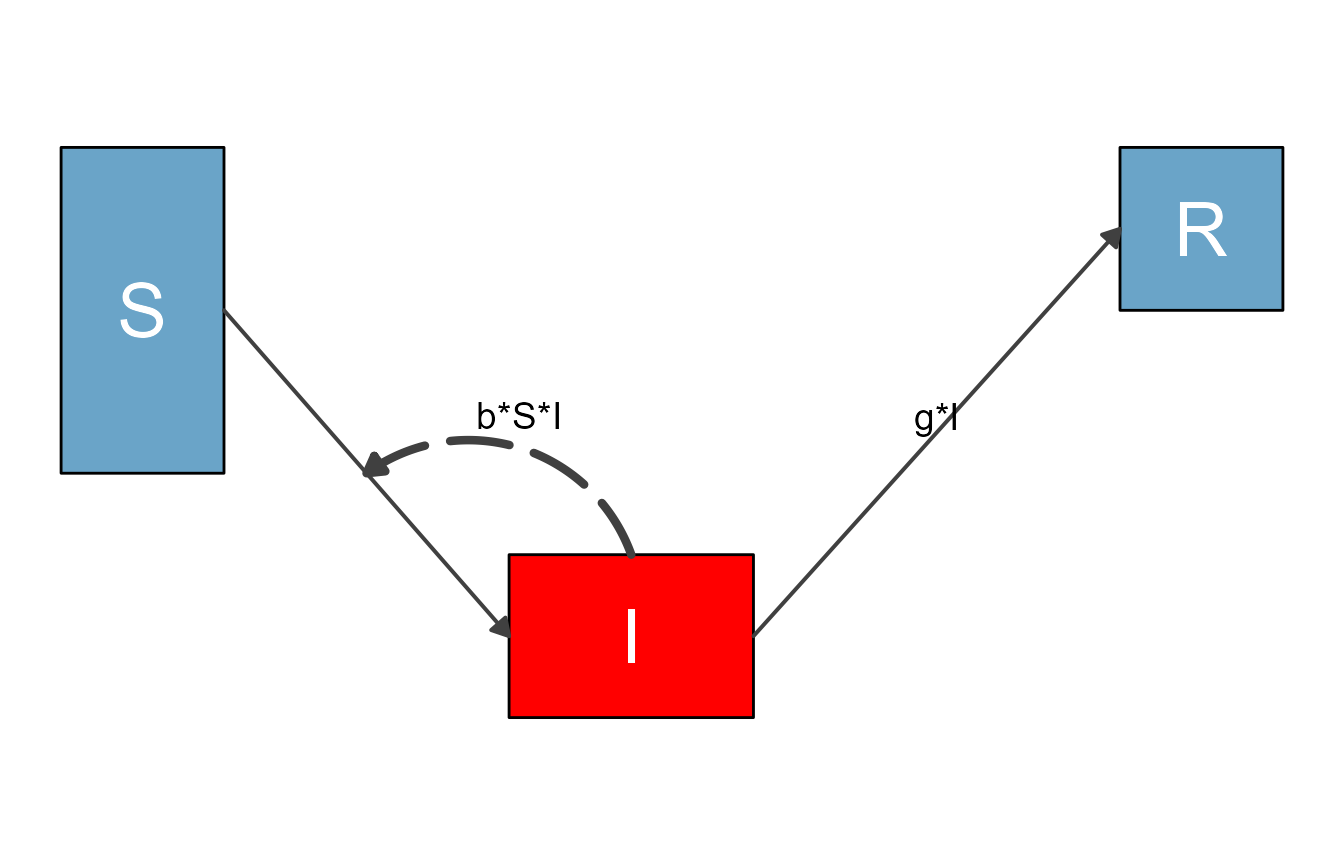When you modify any input with update_diagram(), you generally have two options. You can either specify individual elements you want to update, which we just did. For convenience, there is also a way to update multiple elements at the same time. Let’s say we wanted to update the box colors of all variables and the colors of all main flows, this would do it:

#define updated settings
diagram_settings1 = list(var_fill_color = c(all = "green"),
flow_line_color = c(main = "orange")
)
#update settings
diagram_list4 <- update_diagram(diagram_list1, diagram_settings1)
sir_diagram4 <- make_diagram(diagram_list4)
plot(sir_diagram4)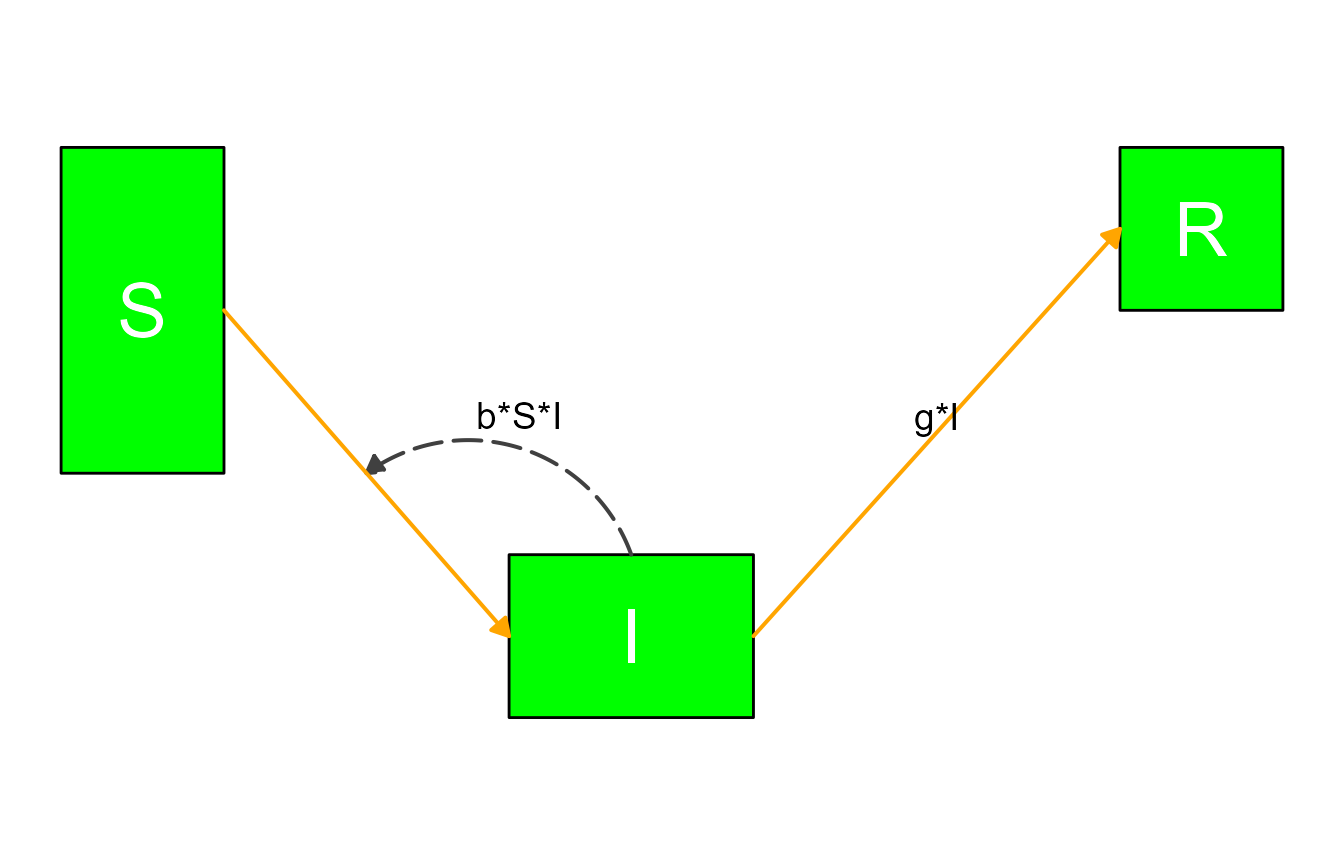The reserved words for update_diagram() are all (for variables or flows) and main, interaction and external (only applicable for flows.)

## Example 2

Next, we are revisiting one of the models discussed in the introductory vignette, the predator-prey model for pathogen and immune response. Here is the model again.

variables = c("Pat","Imm")
flows     = list(Pat_flows = c("g*Pat*(1-Pat/pmax)", "-dP*Pat", "-k*Pat*Imm"),
Imm_flows = c("r*Pat*Imm", "-dI*Imm"))
mymodel = list(variables, flows)

Here is the step that prepares the model, without any optional settings (for now).

diagram_list_v1 <- prepare_diagram(mymodel)

This is how the default plot looks.

diagram_v1 <- make_diagram(diagram_list_v1)
plot(diagram_v1)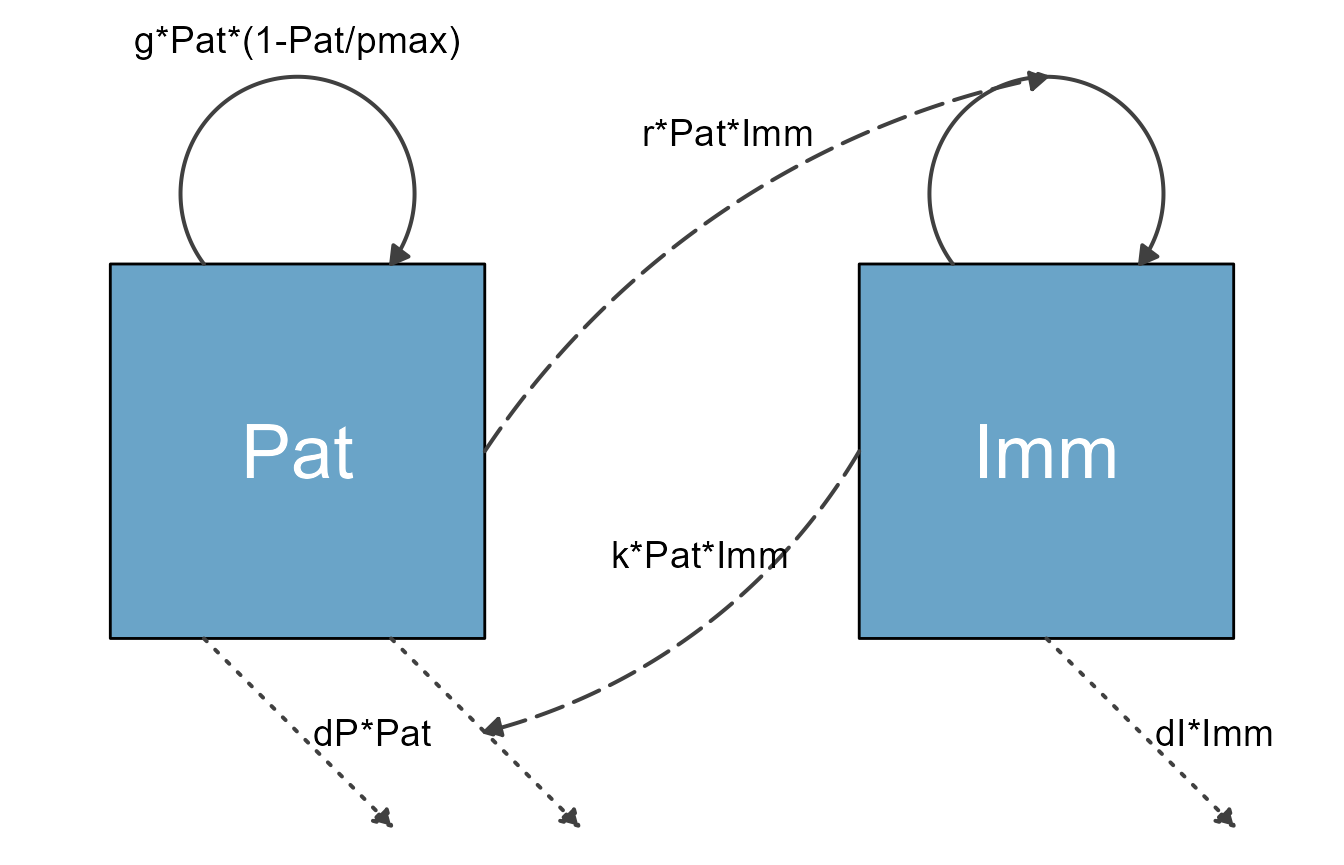Using the update_diagram() function, it is possible to change the look of most components of the diagram. First, we determine the names of the different variables and flows:

update_diagram(diagram_list_v1)
#> No settings were provided; returning names of elements in diagram_list dataframes.
#> Variables: Pat, Imm
#> Flows: m_gPat1Patpmax, m_rPatImm, e_dIImm, e_dPPat, e_kPatImm, i_kPatImm, i_rPatImm

Note that the flow names are simply the equations without the math symbols, and prefaced by type of flow (m = main, i = interaction, e = external). That can make for at times ugly names, but it shouldn’t be much of an issue.

Now we alter the look:

# define new settings
diagram_settings2 = list(var_fill_color = c(Pat = "blue", Imm = "red"),
var_label_color = c(all = "orange"),
flow_line_type = c(main = "dashed"),
flow_line_color = c(m_gPat1Patpmax = "green", m_rPatImm = "orange"),
flow_line_size = c(m_gPat1Patpmax = 1.5, e_dIImm = 2.5, interaction = 3.5),
flow_label_size = c(e_dIImm = 8, external = 2, interaction = 12),
flow_label_color = c(external = "cyan")
)
#update diagram list
diagram_list_v2 <- update_diagram(diagram_list_v1,diagram_settings2)

The result of those modifications is this fairly messy looking diagram:

diagram_v2 <- make_diagram(diagram_list_v2)
plot(diagram_v2)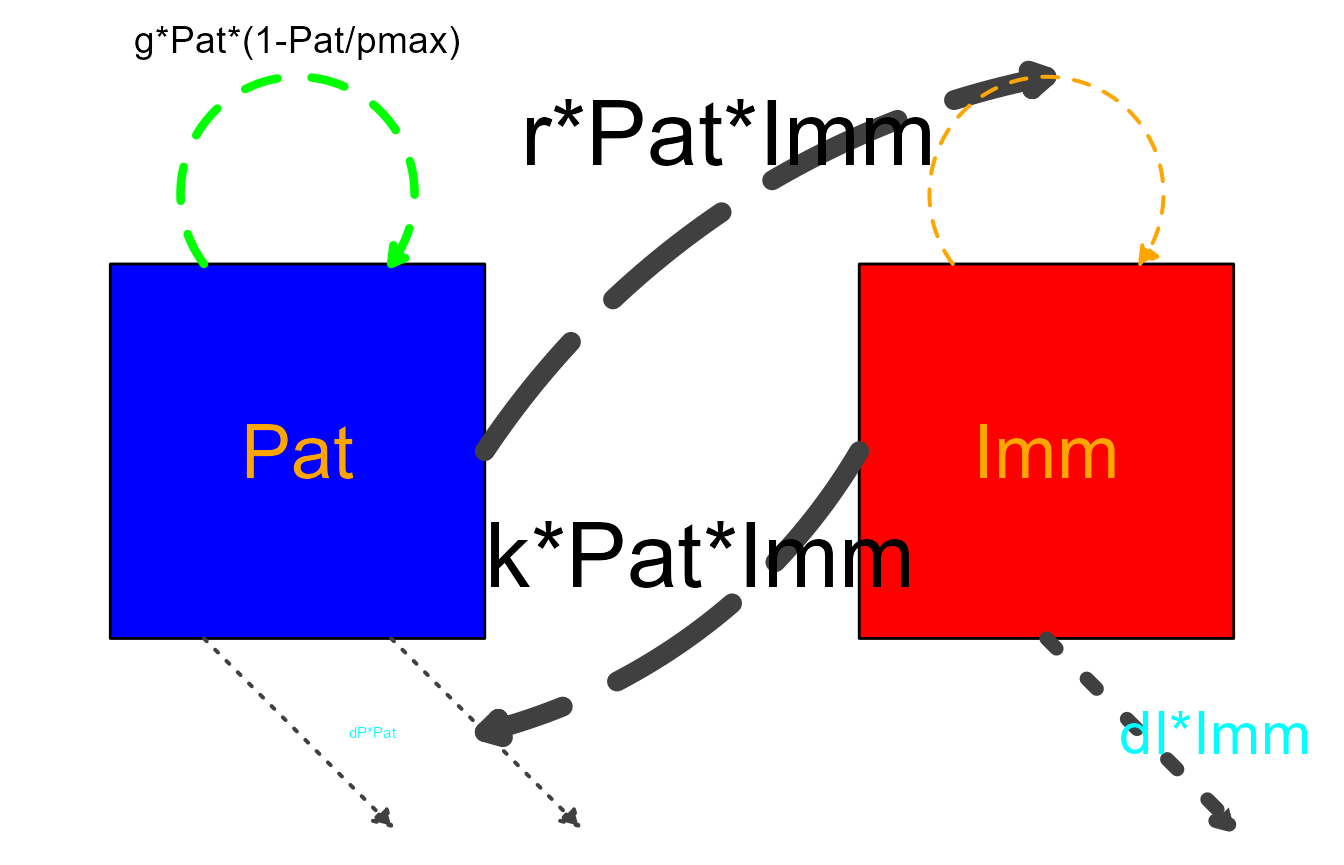Note that for some settings, we used a mix of addressing elements by name and some of the grouping shorthand. If you supply both, the way update_diagram() works is to first process the groupings (all/main/interaction/external) and then any individual ones. This means you can for instance change all boxes to one color and then individually set one to yet another color. We’ll show that in the next example.

## Example 3

Let’s revisit the SIR model with natural births and deaths

# specify the model
variables = c("S","I","R")
flows = list(S_flows = c("n", "-b*S*I", "-m*S"),
I_flows = c("+b*S*I","-g*I", "-m*I"),
R_flows = c("g*I", "-m*R"))
sirmodel = list(variables = variables, flows = flows)

Here are the defaults

#prepare diagram
diagram_list1 <- prepare_diagram(model_list = sirmodel)
#make diagram
sir_diagram1 <- make_diagram(diagram_list = diagram_list1)
#plot diagram
plot(sir_diagram1)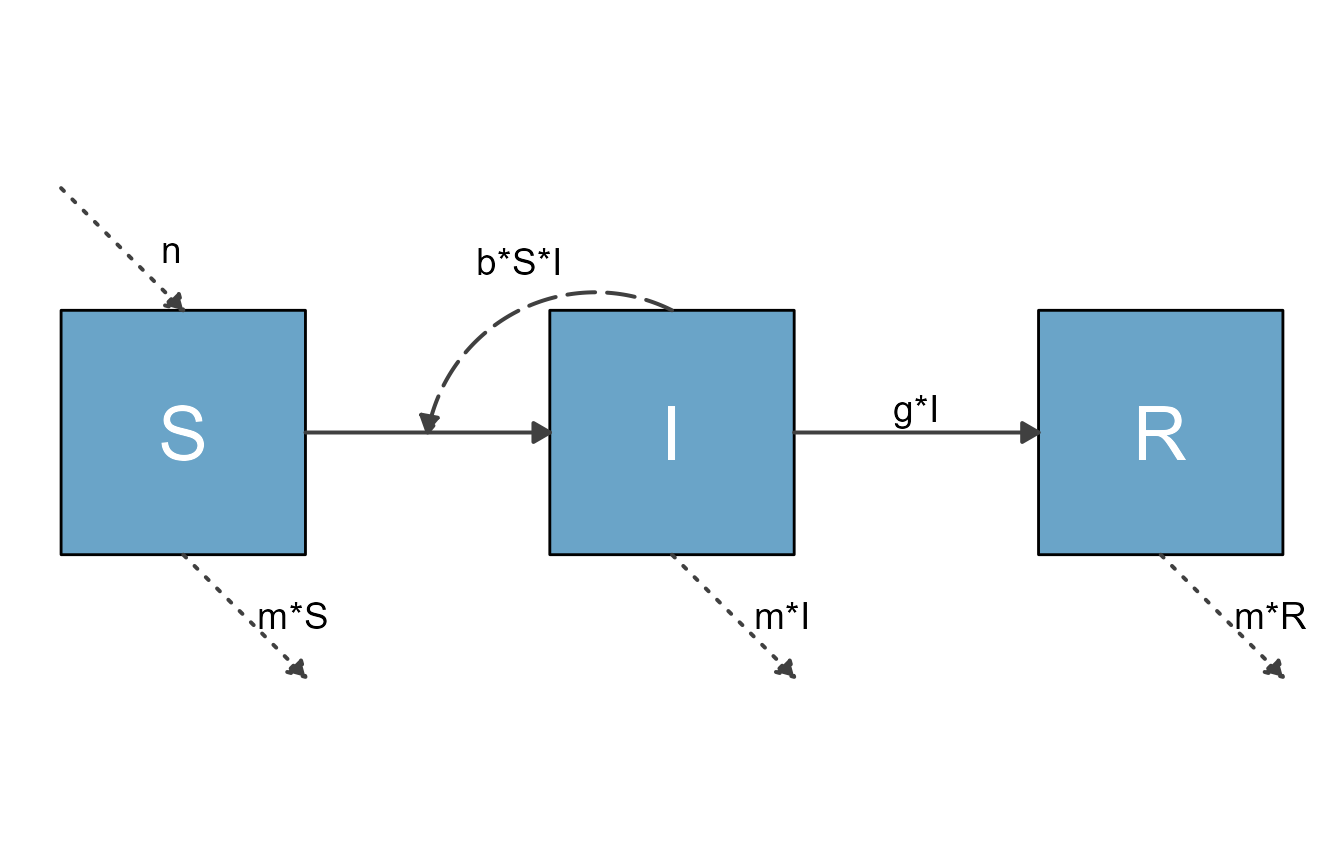Here is an alternative version with layout changes specified through update_diagram().

First, we get the names of all elements:

update_diagram(diagram_list1)
#> No settings were provided; returning names of elements in diagram_list dataframes.
#> Variables: S, I, R
#> Flows: m_gI, m_bSI, e_n, e_mS, e_mI, e_mR, i_bSI

Now we update the look of various elements.

#define new diagram settings outside the update function so we can reuse
diagram_settings = list(
var_fill_color = c(I = "#FFB3A8", all = "#b2b2b2"),
var_outline_color = c(all = "#031e79"),
var_label_color = c(all = "black"),
flow_line_color = c(interaction = "#f94075", external = "blue"),
flow_show_label = c(interaction = FALSE, e_n = FALSE),
flow_show_arrow = c(e_n = FALSE)
)
#update diagram
diagram_list2 <- update_diagram(diagram_list1,diagram_settings)
#make diagram
sir_diagram2 <- make_diagram(diagram_list = diagram_list2)
#plot diagram
plot(sir_diagram2)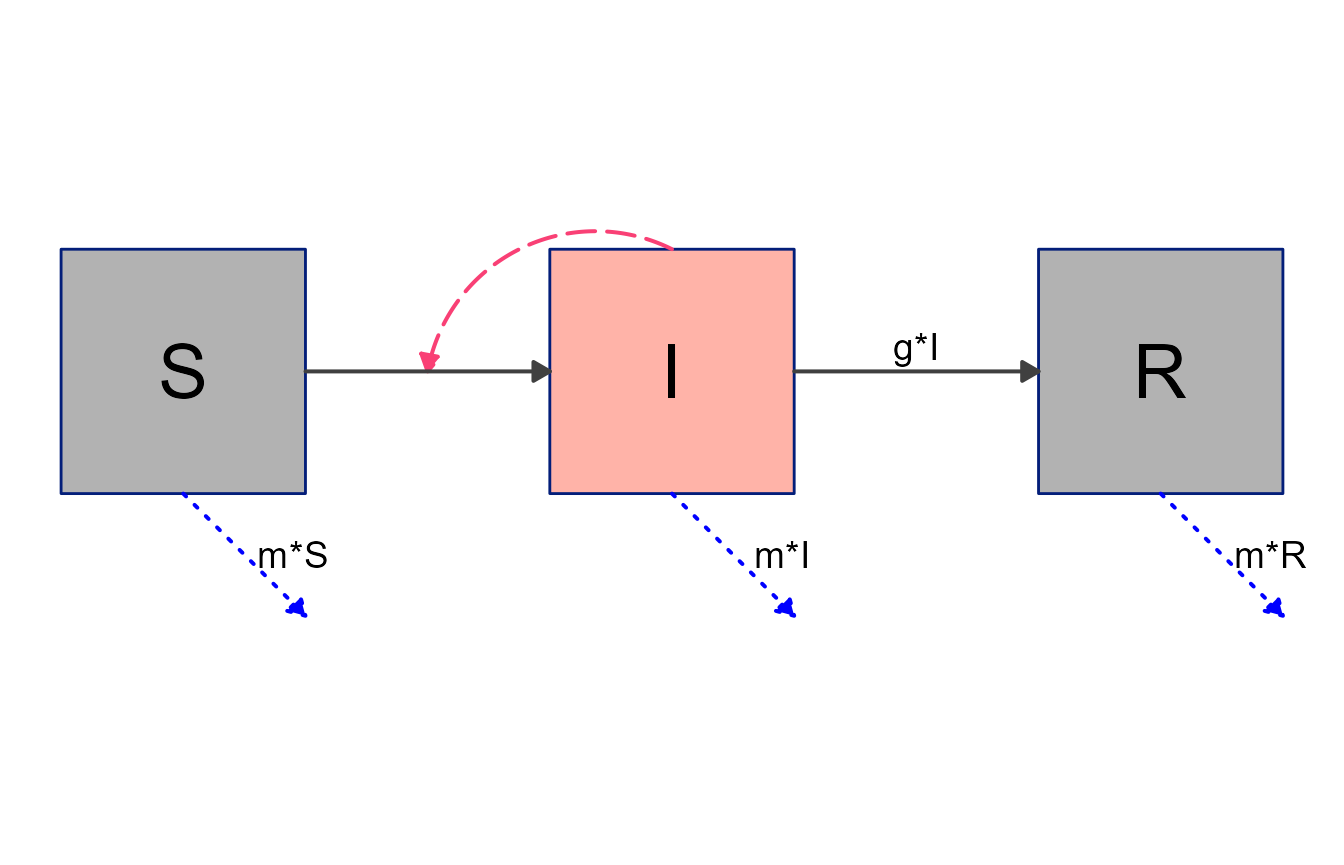Note again the mix of updates applied to individual elements and groups of elements. Groupings are always applied first, no matter the order you supply them. Thus, first we set the color of all boxes to gray, then change the I box individually to red.

## Example 4

It is of course entirely possible to combine layout settings supplied to prepare_diagram() with further styling through update_diagram(). Here is an example, using the SIR model we just explored.

#optional layout settings
sirsettings = list(varlocations = matrix(data = c("S", "","R",
"", "I","" ),
nrow = 2, ncol = 3, byrow = TRUE),
varbox_x_size = c(1, 1.5, 1),
varbox_y_size = c(1, 1, 1),
varspace_x_size = c(1,1),
varspace_y_size = 0.5
)

#prepare diagram
diagram_list3 <- prepare_diagram(model_list = sirmodel, model_settings = sirsettings)
#change styling
diagram_list4 <- update_diagram(diagram_list3,diagram_settings)
#make diagram
sir_diagram4 <- make_diagram(diagram_list = diagram_list4)
#plot diagram
plot(sir_diagram4)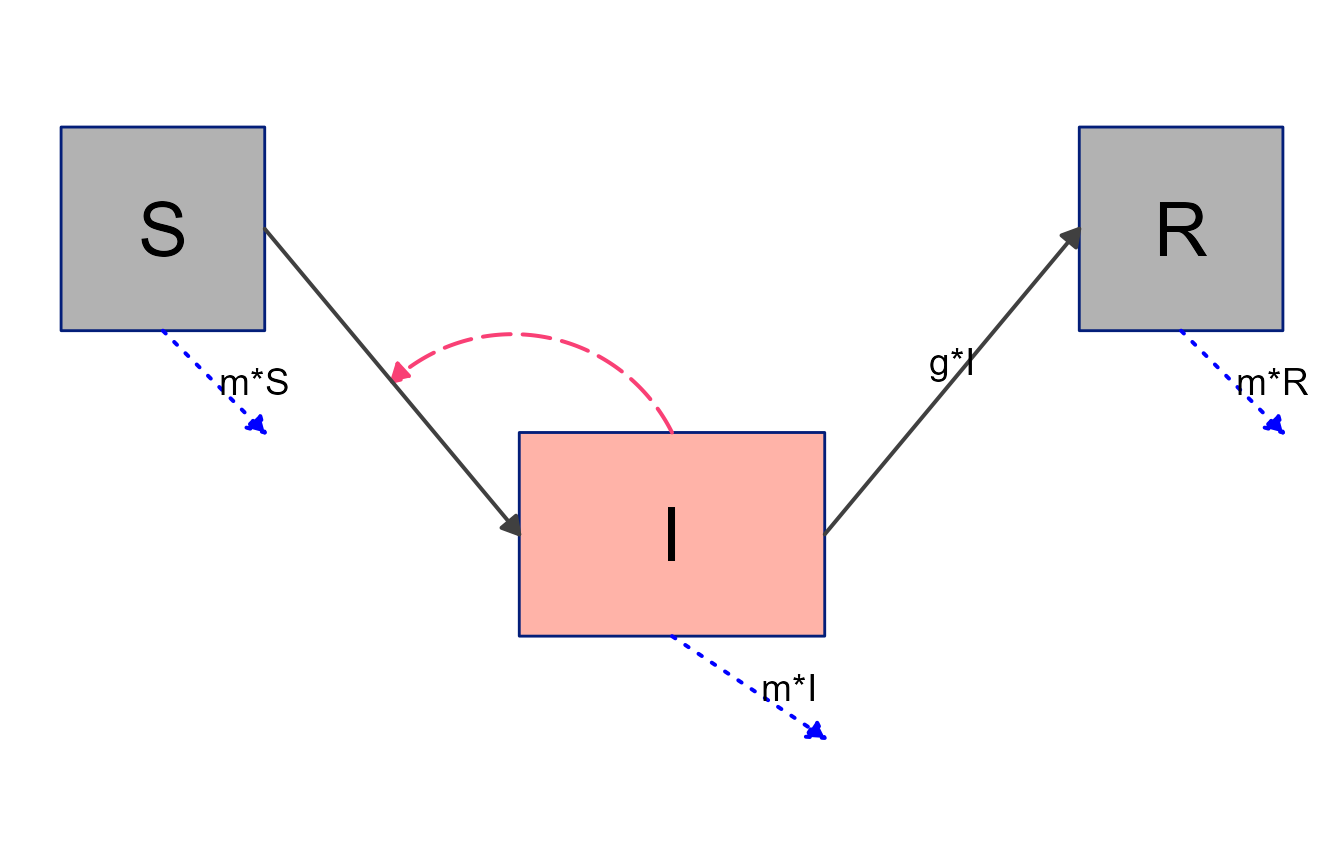## Example 4

Finally, we’ll revisit the 7-compartment model we explored previously, and see if we can get closer to something that could be shown to the public 😄.

variables = c("Sc","Ic","Rc","Sa","Ia","Ra","P")
flows = list(Sc_flows = c("-bcc*Sc*Ic","-bca*Sc*Ia","-bcp*Sc*P"),
Ic_flows = c("bcc*Sc*Ic","bca*Sc*Ia","bcp*Sc*P","-gc*Ic"),
Rc_flows = c("gc*Ic"),
Sa_flows = c("-bac*Sa*Ic","-baa*Sa*Ia","-bap*Sa*P"),
Ia_flows = c("bac*Sa*Ic","baa*Sa*Ia","bap*Sa*P","-ga*Ia"),
Ra_flows = c("ga*Ia"),
P_flows = c("rc*Ic","ra*Ia","-d*P")
)
mymodel = list(variables, flows)
mysettings = list( varlocations = matrix(data = c("Sc", "", "Ic", "Rc",
"",   "P", "",   "",
"Sa", "", "Ia", "Ra"),nrow = 3, byrow = TRUE),
varspace_x_size = 2,
varspace_y_size = 1
)

Prepare the diagram with additional settings.

diagram_list5 <- prepare_diagram(mymodel, mysettings)

Update look of diagram

#get element names
update_diagram(diagram_list5)
#> No settings were provided; returning names of elements in diagram_list dataframes.
#> Variables: Sc, Ic, Rc, Sa, Ia, Ra, P
#> Flows: m_gcIc, m_gaIa, m_bccScIc, m_bacSaIc, e_dP, i_rcIc, i_raIa, i_bccScIc, i_bcaScIa, i_bcpScP, i_bacSaIc, i_baaSaIa, i_bapSaP
# define list of updates
diagram_settings2 = list(var_fill_color = c(Sc = "#cde9fa", Ic = "#cde9fa",
Rc = "#cde9fa", Sa = "#40f9cf",
Ia = "#40f9cf", Ra = "#40f9cf",
P = "#b2b2b2"),
var_outline_color = c(all = "#031e79"),
var_label_size = c(all = 8),
var_label_color = c(all = "black"),
flow_line_color = c( interaction = "#f94075",
external = "gray")
)
diagram_list6 <- update_diagram(diagram_list5, diagram_settings2) 

Now we make the diagram and plot it.

model_plot6 <- make_diagram(diagram_list6)
plot(model_plot6)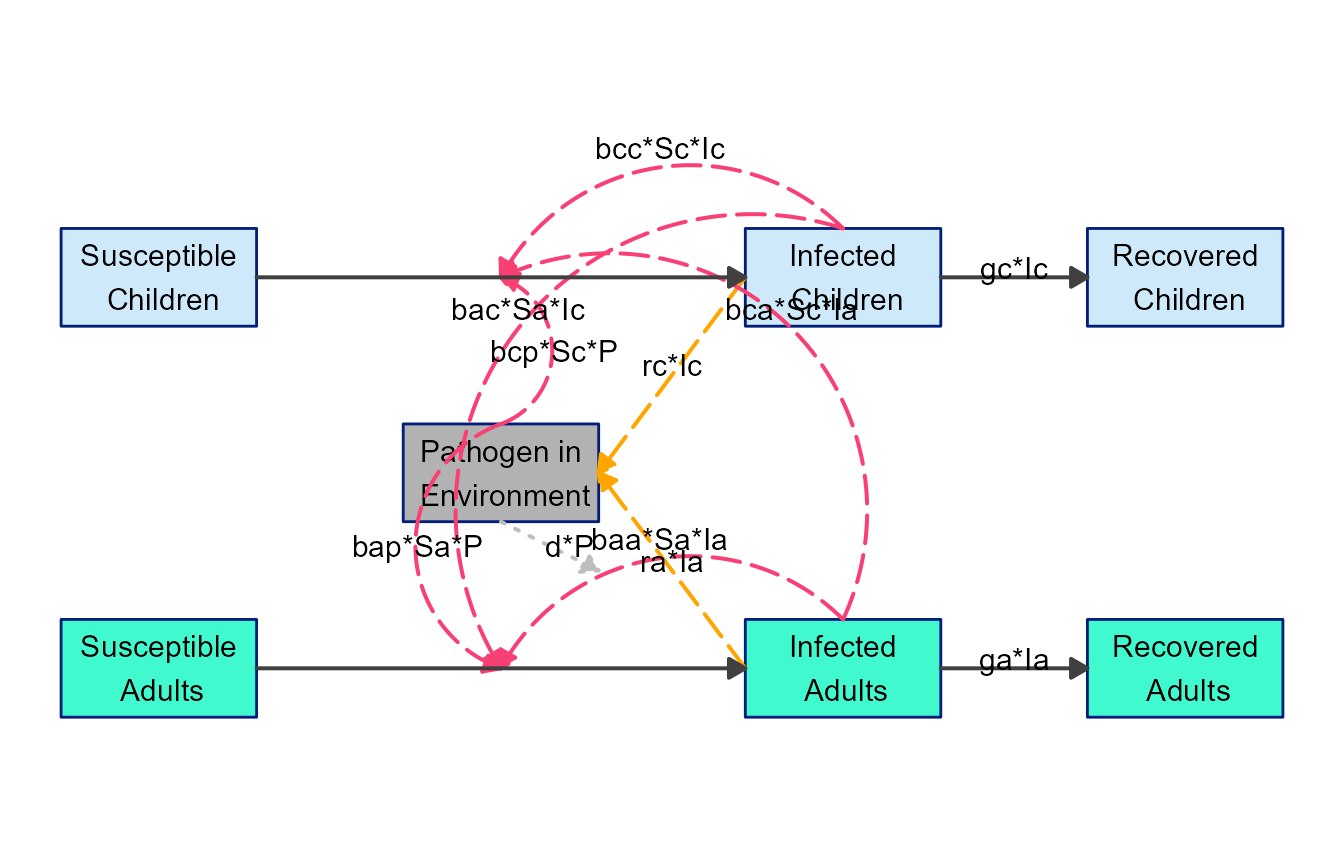Better, but not quite there. One can use update_diagram() more than once of course, so let’s make further changes. Specifically, we’ll modify some of the locations for the arrows and labels.

The way update_diagram() processes any change to location of boxes, arrows, labels, etc. is by applying a change relative to the current position. This means if you think a box for some variable should be extended a bit to the left, you can apply an offset of say -0.25 to var_xmin for that variable. Similarly, if you think the box should be extended upwards a bit, you can apply 0.25 to the var_ymax value. As you adjust positioning, it might be helpful to turn on the grid lines in the diagram, which are by default turned off. You can do so by setting with_grid = TRUE in make_diagram().

Here is a simple example where we moved a few of the labels

# define list of updates
diagram_settings3 = list(flow_ylabel = c(m_gcIc = 0.25, m_gaIa = 0.25) )
diagram_list7 <- update_diagram(diagram_list6, diagram_settings3)
model_plot7 <- make_diagram(diagram_list7, with_grid = TRUE)
plot(model_plot7)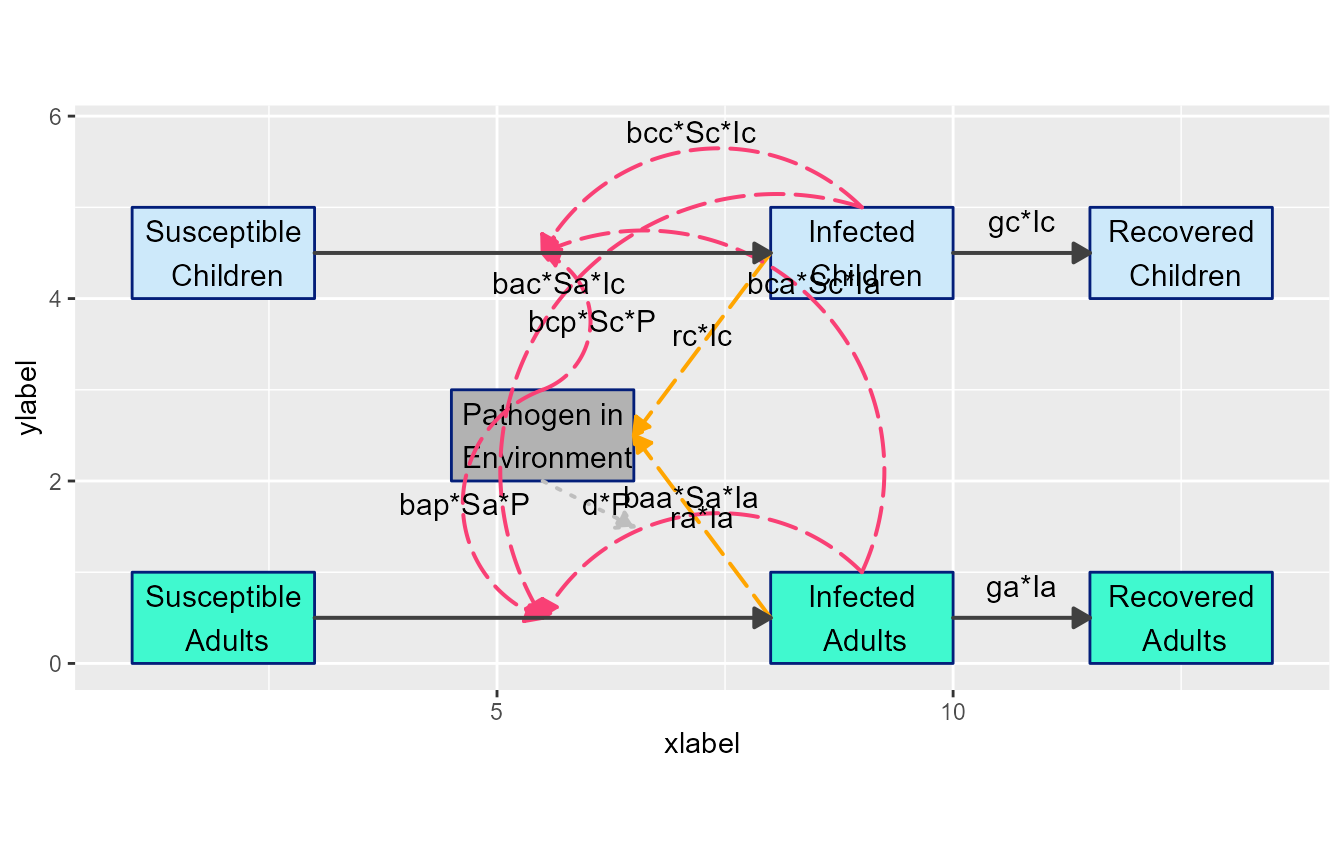Next, let’s move some arrows around to make things look less messy. Note that start and end points for arrows are specified as relative to the current value, however curvature is an absolute value. Positive values curve in one direction, negative in the other, and 0 means no curvature. We are also changing a few flow colors to make things clearer.

# define list of updates
diagram_settings4 = list(flow_curvature = c(i_baaSaIa = -0.5, i_bccScIc = 0.5,
i_bacSaIc = -0.3, i_bcaScIa = 0.3,
i_raIa = 0, i_rcIc = 0, i_bcpScP = -0.5),
flow_xstart = c(i_bcpScP = -0.5, i_bapSaP = -0.5),
flow_ystart = c(i_bcpScP = -0.5, i_bapSaP = -0.5, i_bacSaIc = -1),
flow_line_color = c(i_baaSaIa = "#40f9cf", i_bacSaIc = "#cde9fa",
i_bapSaP = "orange", i_bcpScP = "orange",
i_bccScIc = "#cde9fa", i_bcaScIa = "#40f9cf"),
flow_line_size = c(all = 1.25)
)
diagram_list8 <- update_diagram(diagram_list7, diagram_settings4)
model_plot8 <- make_diagram(diagram_list8, with_grid = TRUE)
plot(model_plot8)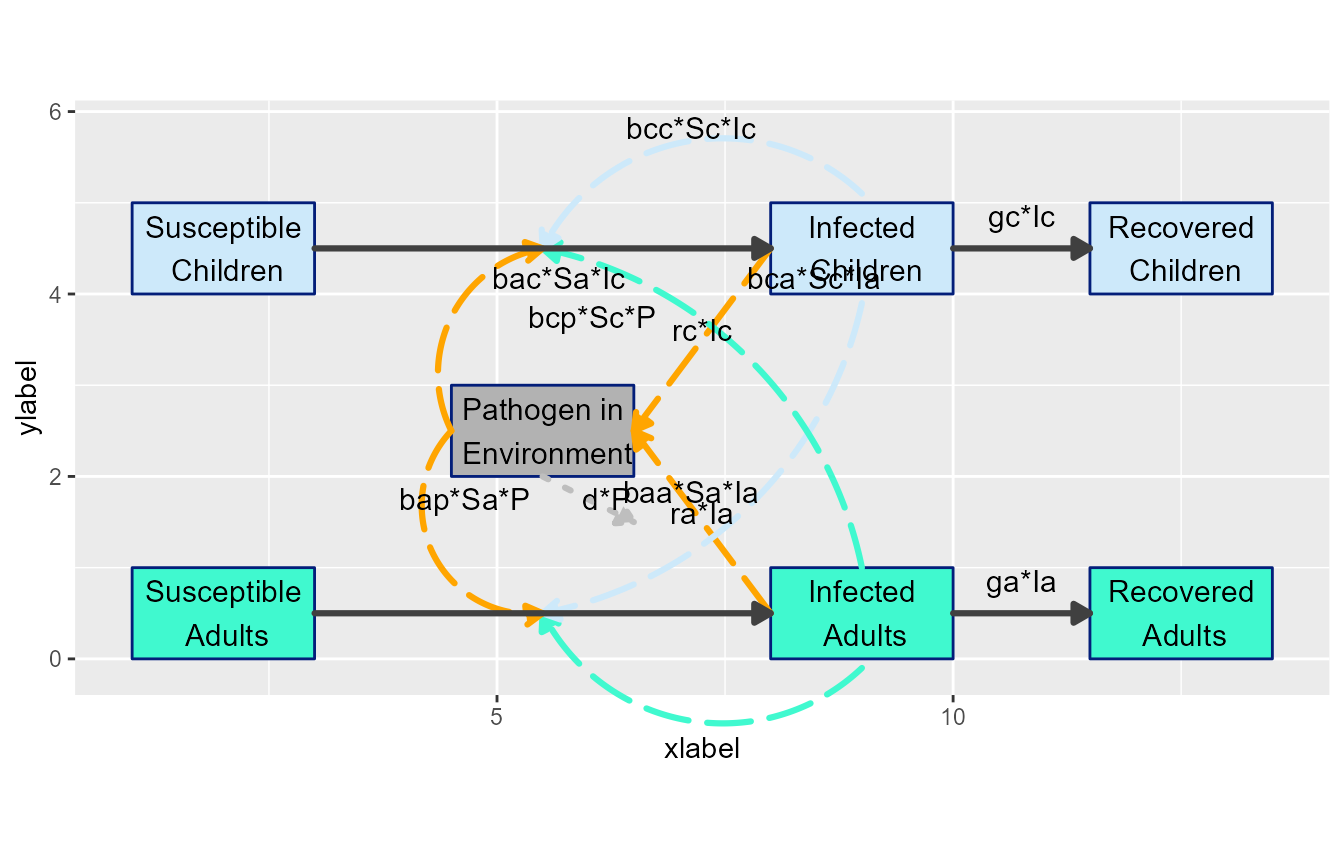Now we need to adjust the labels

# define list of updates
diagram_settings5 = list(flow_xlabel = c(i_bcaScIa = 0.75, i_bacSaIc = 3.5,
i_bapSaP = -0.75, i_bcpScP = -2,
i_raIa = -0.25, i_rcIc = 0.5),
flow_ylabel = c(i_bcaScIa = -2.5, i_bacSaIc = -0.25,
i_baaSaIa = -2, e_dP = -0.5,
i_rcIc = -0.75)
)
diagram_list9 <- update_diagram(diagram_list8, diagram_settings5)
model_plot9 <- make_diagram(diagram_list9, with_grid = FALSE)
plot(model_plot9)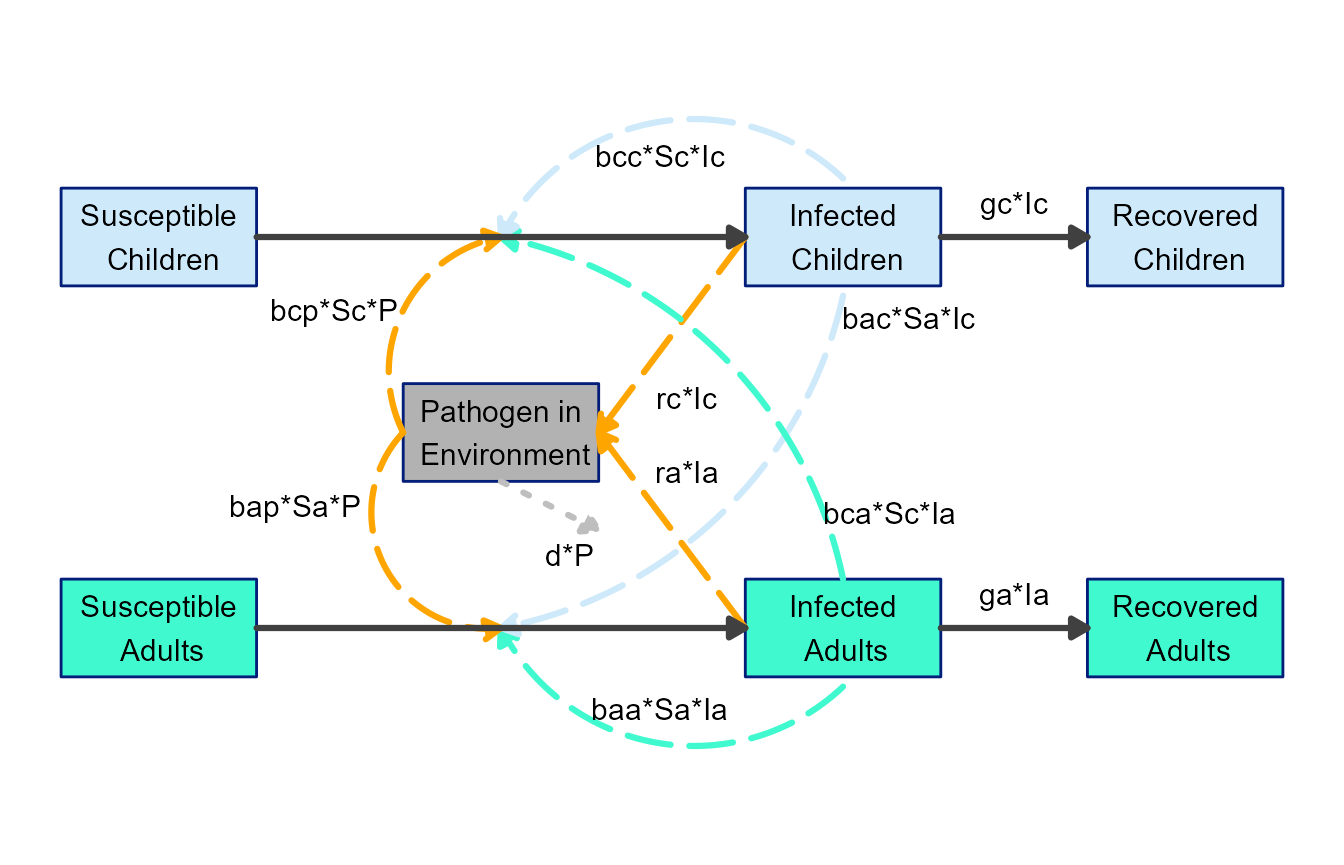This is now looking like a pretty decent diagram. With maybe a few more further tweaks, this could be used for public consumption 😁. Granted, adjusting the arrows and labels can be a bit fiddly, but we think it’s still easier and better than firing up your favorite drawing software. Also, it’s likely easier to update here if you make changes to the model.

## Next steps

By combining layout settings supplied to prepare_diagram() and styling applied through update_diagram(), you can easily customize the look of the diagram. Often, this should allow you to produce a diagram that looks ready to use.

In those instances where you want to make further changes that are not currently supported by this workflow (e.g., if you want to change one of the variable boxes to a circle), flowdiagramr can provide you with the complete code that generates the ggplot object, which you can then edit manually. The next vignette explains how to do that.